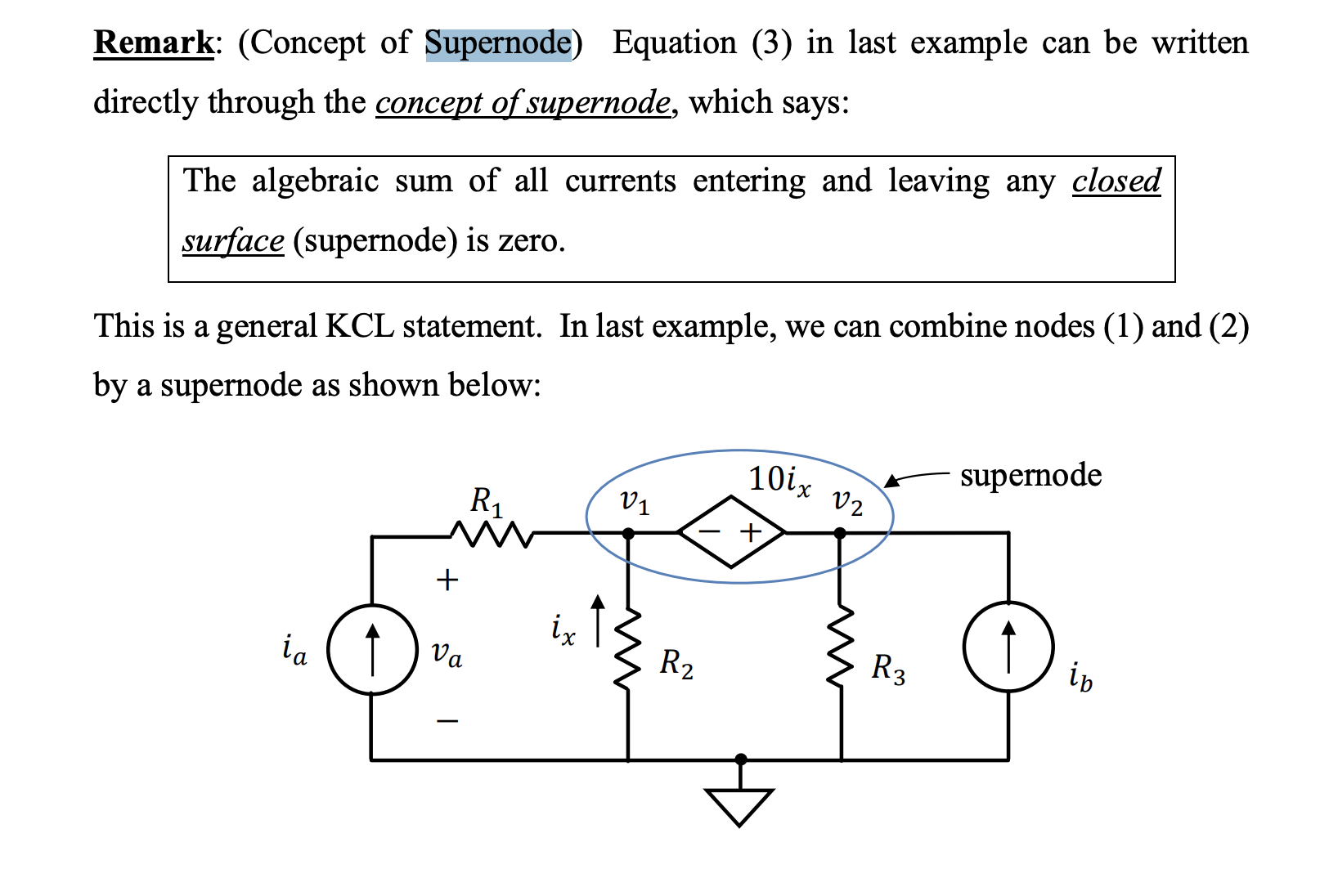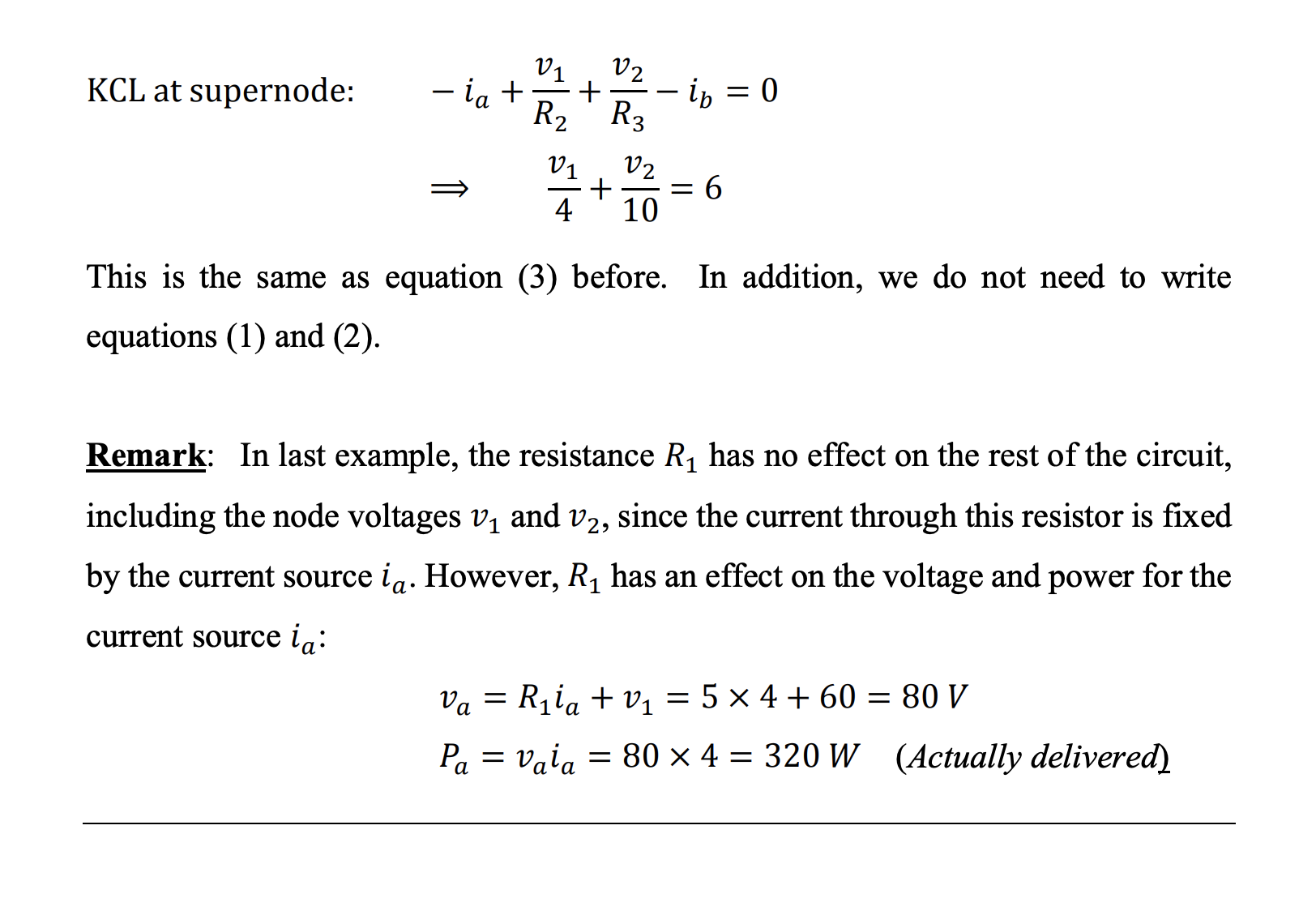# Node-Voltage Analysis §

Always assume that the current is leaving the node unless otherwise indicated.

Leaving currents will be taken as **positive (+)** while entering currents are taken to be **negative (-)** in KCL equations.

### Terminology §

Essential Node: A node where three or more circuits elements are connected.

Reference Node: One of the essential nodes. Chosen arbitrarily, and assumed to have zero-voltage.

Labeled ->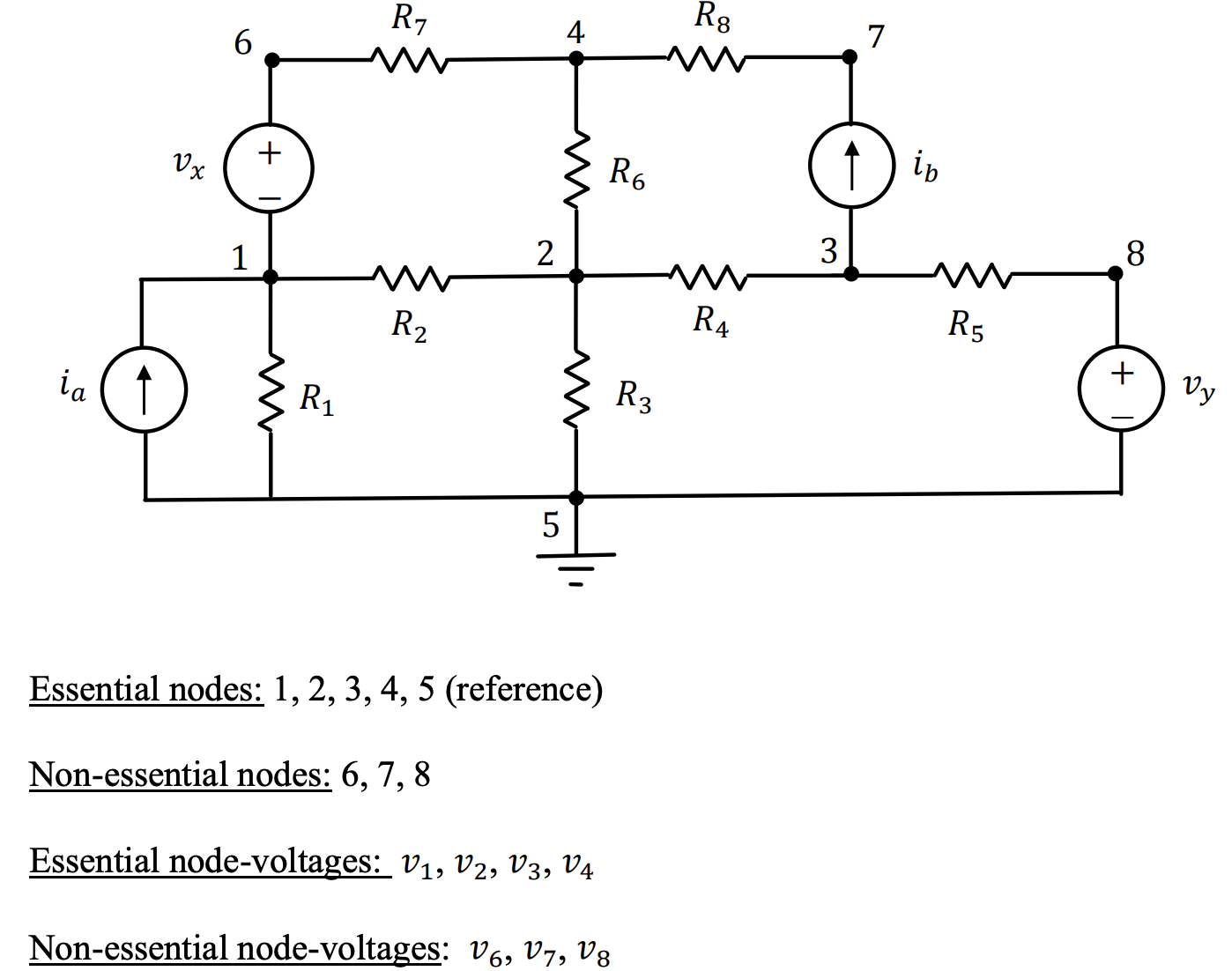Node-Voltage: The voltage-drop from a non-reference node to the reference node.

Essential Node-Voltage: The voltage-drop from a non-reference essential node to the reference node.

### Procedure §

1. Choose a reference node and label (N-1) node-voltages
2. Assign currents to voltages (optional)
3. Apply [[notes/Kirchhoff’s Laws#Kirchhoff’s Junction Current Law KCL|Kirchhoff’s Laws#Kirchhoff’s Junction Current Law KCL]] at each non-reference essential node to get (N-1) equations
4. Use Ohm’s Law and Ohm’s Law and [[notes/Kirchhoff’s Laws#Kirchhoff’s Loop Voltage Law KVL|Kirchhoff’s Laws#Kirchhoff’s Loop Voltage Law KVL]] to express the currents in terms of the node-voltages.
5. Solve the resulting (N-1) linear independent equations for the (N-1) unknown node-voltages.

### Example §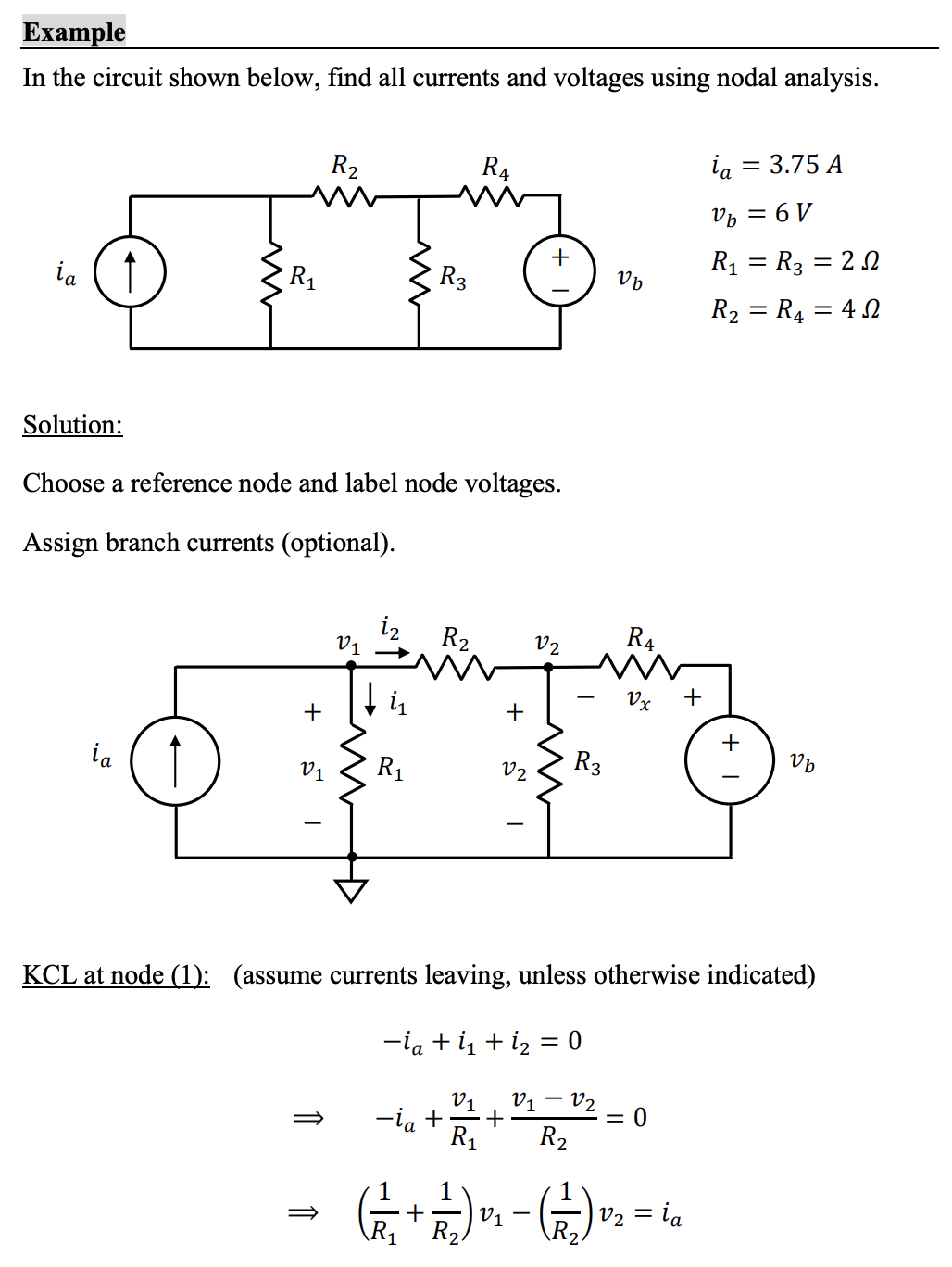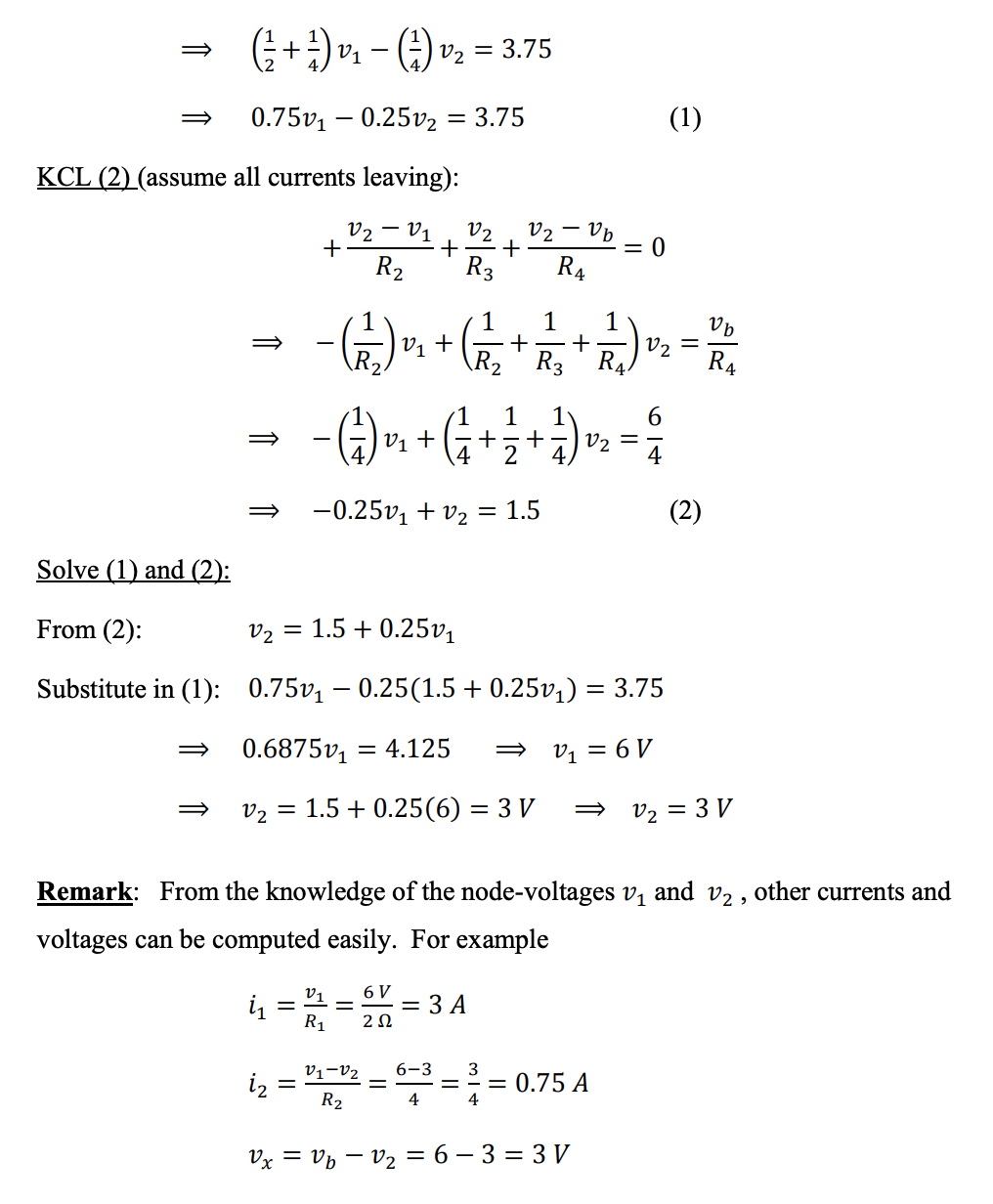To calculate , always do positive side of voltage minus negative side. Example: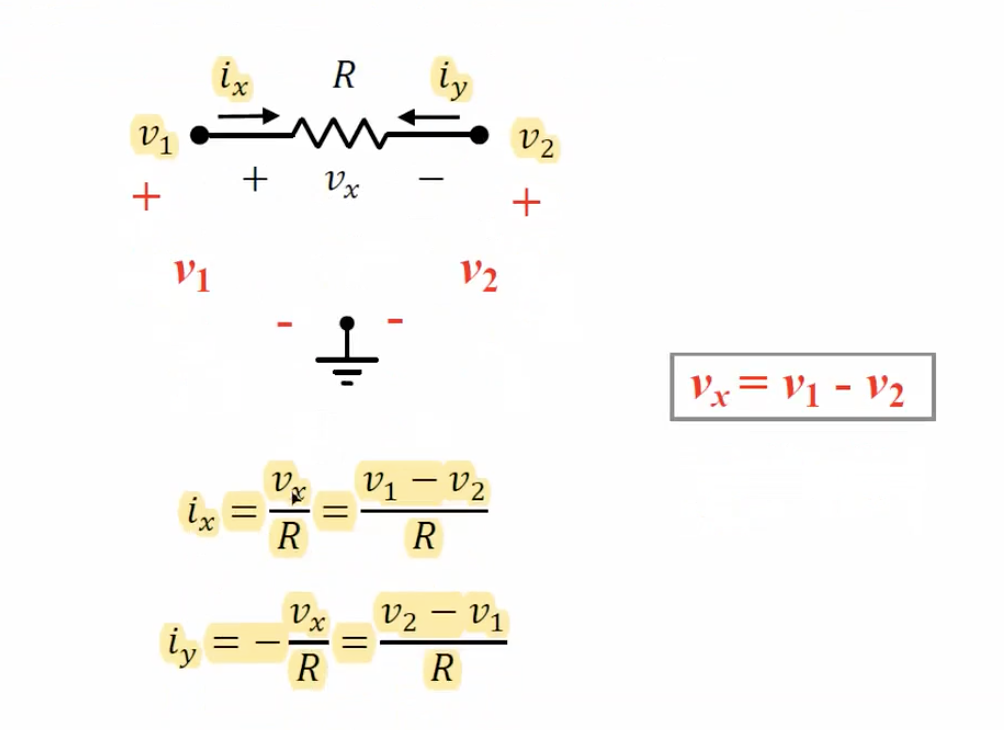### Three-Points Rule §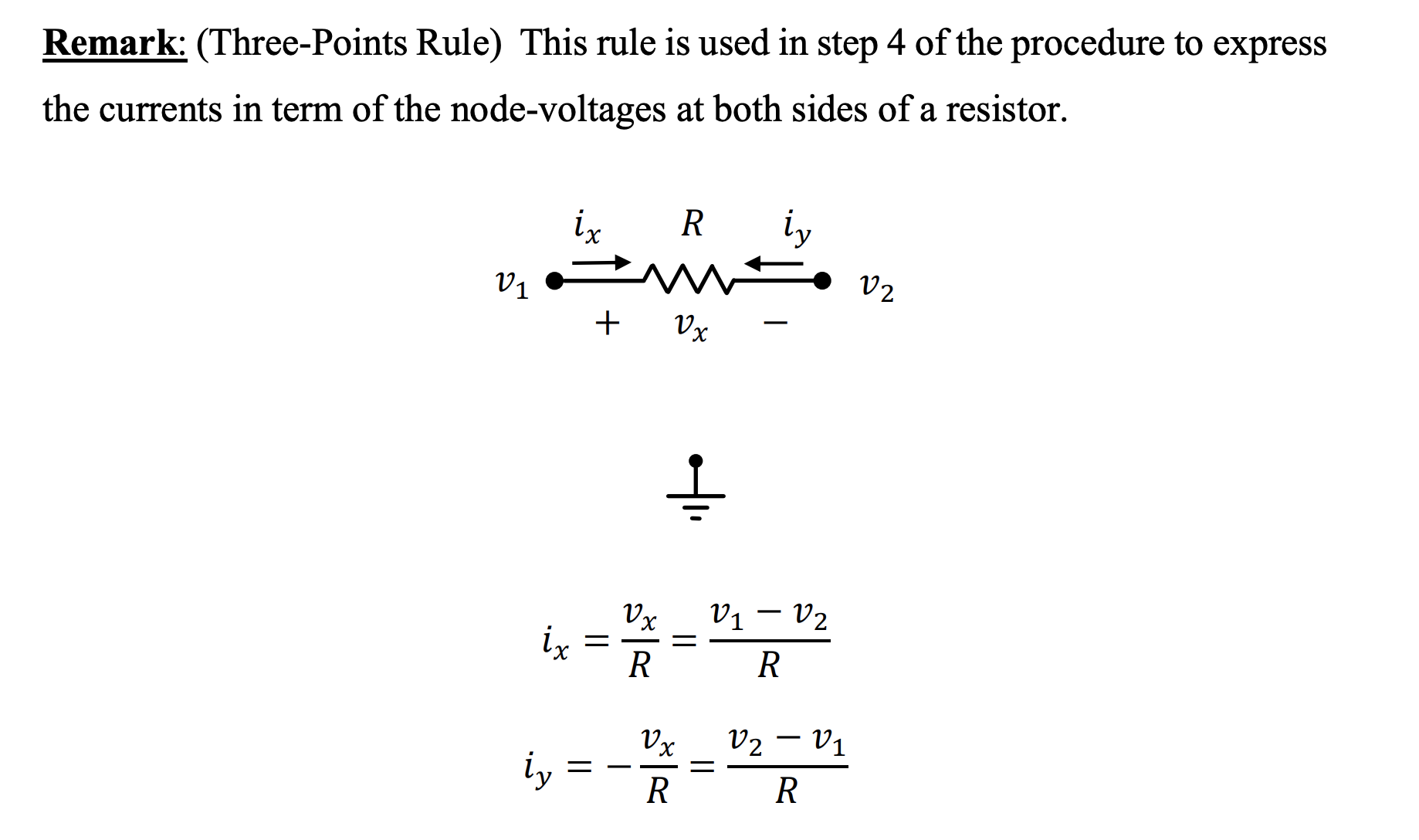### Supernode Analysis §

You can simply ignore the voltage source between two nodes.